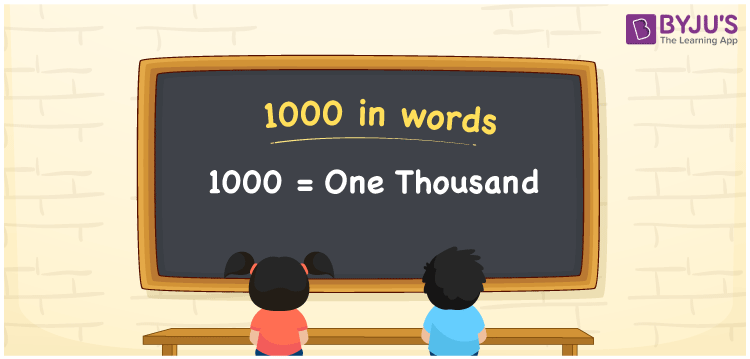# 1000 in Words

We can write 1000 in words as One thousand. Sometimes 1000 can also be written in words as Ten hundred. We can convert 1000 into words with the help of a place value chart effortlessly. If you donated Rs. 1000 to an old woman, you can say, “I donated One thousand rupees to an old woman”. Also, note that 1000 is a cardinal number as it conveys a distinct quantity.

 1000 in words Thousand or One thousand Thousand in Numerical Form 1000

## 1000 in English Words

We generally write numbers in words using the English alphabet. Thus, we can read 1000 in English as “One thousand”.## How to Write 1000 in Words?

As mentioned above, in this section, you will learn how to write 1000 in words using a place value chart. The number 1000 has 4 digits, so we need to make a chart that contains the place value up to 4 digits as shown below.

 Thousands Hundreds Tens Ones 1 0 0 0

Thus, we can write the expanded form as:

1 × Thousand + 0 × Hundred + 0 × Ten + 0 × One

= 1 × 1000 + 0 × 100 + 0 × 10 + 0 × 1

= 1000

= One thousand

Therefore, 1000 in words is written as One thousand.

1000 is a natural number that precedes 1001 and succeeds 999.

1000 in words – One thousand

Is 1000 an odd number? – No

Is 1000 an even number? – Yes

Is 1000 a perfect square number? – No

Is 1000 a perfect cube number? – Yes

What is the cube root of 1000? – 10

Is 1000 a prime number? – No

Is 1000 a composite number? – Yes

## Frequently Asked Questions on 1000 in Words

Q1

### How do you write 1000 in words?

We can write 1000 in words as One thousand.
Q2

### What is the English word for 1000?

The English word to represent the number 1000 is “a thousand” or “one thousand”.
Q3

### How do you write 1000 in the form of words for a cheque?

We generally write 1000 in words as One Thousand rupees only, for a cheque.
Test your Knowledge on 1000 in Words